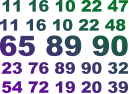# Comprehensive Online Math Toolkit

## Math

Mathematics is a vast and complex field of study, with many different branches. Some of the most common branches of mathematics include:
• Arithmetic is a separate branch of mathematics. It is the study of numbers and the basic operations of addition, subtraction, multiplication, and division. Arithmetic is the foundation for many other branches of mathematics, such as algebra, geometry, and calculus.
• Algebra: Algebra is the study of symbols and the rules for manipulating them. It is used in many different areas of mathematics, including geometry, calculus, and statistics.
• Geometry: Geometry is the study of shapes and space. It is used in many different areas of mathematics, including trigonometry, calculus, and topology.
• Statistics: Statistics is the study of data and how to collect, analyze, and interpret it. It is used in many different areas of science, business, and government.
• Calculus: Calculus is the study of change and motion. It is used in many different areas of mathematics, including physics, engineering, and economics.
• Number theory: Number theory is the study of the properties of numbers. It is one of the oldest and most well-studied branches of mathematics.
• Topology: Topology is the study of the properties of shapes that are preserved under continuous deformations. It is used in many different areas of mathematics, including geometry, differential geometry, and algebraic geometry.
In addition to these main branches, there are many other branches of mathematics, such as discrete mathematics, mathematical logic, and computational mathematics.
Below, we have covered modules that largely comes under Arithmetic and rest under Geometry and Statistics.